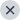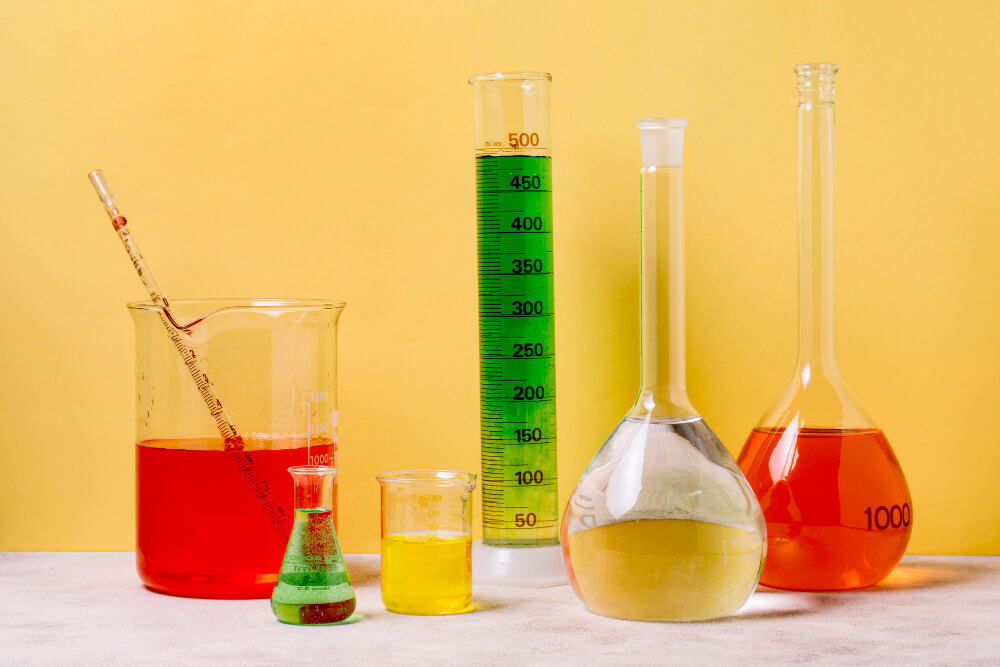013 July, 2023 By Artika Shah, IMS

GATE Syllabus for Chemical Engineering is very important for achieving good marks in the Graduate Aptitude Test in Engineering.  It contains topics needed for the exam and questions from this prescribed syllabus that will be included in the question paper. IISc Bangalore will release the GATE 2024 Syllabus for Chemical Engineering.

Sections of the GATE Syllabus for Chemical Engineering

The GATE Chemical Engineering (CH) Syllabus is divided into nine main sections including:
1. Process Calculations and Thermodynamics
2. Engineering Mathematics
3. Heat Transfer
4. Mass Transfer
5. Mechanical Operations and Fluid Mechanics
6. Chemical Reaction Engineering
7. Plant Design and Economics
8. Instrumentation and Process Control
9. Chemical Technology

Below are the key sections of the GATE 2024 Syllabus for Chemical Engineering:

1. Process Calculations and Thermodynamics

The process calculations and Thermodynamics section of the GATE syllabus for Chemical Engineering cover key topics like moar properties, phase equilibria, and laws of entropy.

Some of the key topics covered include:
1. Steady and unsteady state mass and energy balances include multi-component, multi-phase, reacting, and non-reacting systems. Other topics covered are the use of tie components, recycle, bypass, and purge calculations, Gibbs phase rule, and degree of freedom analysis.
2. First and Second laws of thermodynamics. The applications of the first law to close and open systems. Second Law and Entropy. Thermodynamic properties of pure substances. Equation of state and residual properties of mixtures. Partial molar properties, fugacity, excess properties, and activity coefficients, predicting VLE of systems, and chemical reaction equilibrium.

Engineering Mathematics Syllabus
GATE Chemical Engineering (CH) Syllabus for the Engineering Mathematics section encompasses topics including probability, calculus, and numerical methods.

Let us discuss each topic in more detail:
1. Linear Algebra: Eigenvectors, Eigenvalues, Systems of linear equations, &  matrix algebra.

2. Calculus: The topics under this section include the function of a single variable, continuity, and differentiability, limit, mean value theorems, Taylor series, partial derivatives, evaluation of definite and improper integrals, maxima and minima, total derivatives, divergence and curl, gradient, vector identities, directional derivatives, line, surface and volume integrals, stokes, Green’s and Gauss theorems.

3. Complex Variables: This section consists of topics like a polar form of a complex number, complex number, and triangle equality. The probability and statistics section includes topics including definitions of probability and sampling theorems, conditional probability, mean, median, mode and standard deviation, Poisson, random variables, linear regression analysis, and binomial distributions.

4. Differential Equations: This sections includes topics like the first-order equations (linear and nonlinear), Cauchy’s and Euler’s equations, higher-order linear differential equations with constant coefficients, Laplace transforms, initial and boundary value problems, and solutions of one-dimensional heat and wave equations.

5. Numerical Methods: The topics covered include numerical solutions of linear and non-linear algebraic equations. Other topics are integration by trapezoidal and Simpson’s rule and single and multi-step methods for numerical solutions for different equations.

Heat Transfer
This section includes topics like heat exchangers and condensation and evaporators. Some other topics are convection and radiation, equation of energy, steady and unsteady heat conduction, types of heat exchangers and evaporators and their process calculations, thermal boundary layer and heat transfer coefficients, condensation, and evaporation, boiling,  design of double pipe, shell and tube heat exchangers and single and multiple effect evaporators.

Fluid Mechanics and Mechanical Operations Syllabus
GATE Chemical Engineering (CH) Syllabus for Fluid Mechanics and Mechanical Operations covers topics such as flow metering, dimensional analysis, and turbulent flow.

The topics are mentioned in detail below:

1. Partial size and shape, free and hindered settling, particle size distribution, size reduction and classification, cyclones and centrifuge, thickening and classification, agitation, filtration and mixing, and conveying of solids.

2. Shell balances include a differential form of the Bernoulli equation and energy balance, fluid statistics, surface tension, Newtonian and non-Newtonian fluids, equation of motion, and equation of continuity.

3. Equation of macroscopic friction factors, equation of mechanical energy, dimensional analysis and similitude, velocity profiles, flow through pipeline systems, pumps, and compressors, flow meters, elementary boundary layer theory, flow past immersed bodies including packed and fluidized beds, Turbulent flow: Universal velocity, fluctuating velocity profile and pressure drop.

4. Particle size and shape, size reduction and classification of solid particles, particle size distribution, centrifuge, and cyclones, free and hindered settling, filtration, thickening and classification, mixing, agitation, and conveying of solids.

Mass Transfer
GATE Chemical Engineering (CH) Syllabus for the mass transfer section includes topics like momentum, molecular diffusions, and heat mass transfer.

Below are topics mentioned in detail:

• Molecular diffusion in Fluids
• Fick’s law
• Momentum, Heat, and mass transfer analogies
• Mass transfer coefficients, penetration, film, and surface renewal theories
• HTU and NTU concepts
• Stage-wise and continuous contacting and stage efficiencies
• Design and Operation of Equipment for absorption, distillation, liquid-liquid extraction, leaching, humidification, drying, adsorption and dehumidification, and membrane separations (Ultra-filtration, micro-filtration, nano-filtration and reverse osmosis.)

Instrumentation and Control
GATE Chemical Engineering (CH) Syllabus for the instrumentation and process control section includes topics like control valves, transducer dynamics, and process reaction valves.
Below are
the topics mentioned in detail:

• P & ID equipment symbols
• Measurement of process variables, transducers, and sensors
• Control valves
• Linearization and process modeling, transfer functions and dynamic responses of various systems, process reaction curves, systems with the inverse response and controller modes (PI, P, and PID).
• Transducer Dynamics
• Control Valves
• Analysis of closed-loop systems, including, controller tuning, stability, frequency response, feedforward control, and cascade.

Plant Design and Economics
GATE Chemical Engineering (CH) Syllabus for the Plant Design and Economics section includes topics such as discounted cash flow, payback period, and cost indices

The topics covered in this section include:

• Principles of process economics and cost estimation consist of depreciation and total annualized cost, rate of return, cost indices, discounted cash flow, payback period, optimization in process design, and sizing of the chemical engineering equipment such as multi-stage contactors and heat exchangers.

Chemical Technology
GATE Chemical Engineering (CH) Syllabus for Chemical Technology encompasses topics like fertilizers, petrochemicals, and natural products.

Each topic is discussed in detail:
1. Natural products industries (Sugar, Oil, Pulp and Paper, and Fats).
2. In organic chemical industries (Phosphoric Acid, Sulphuric Acid, and Chlor-Alkali industry) and fertilizers (Urea, SSP, TSP, and Ammonia)
3. Petrochemicals and Petroleum Refining.
4. Polymerization Industries ( PVC, Polypropylene, Polyethylene, and polyester synthetic fibers).

GATE 2024 Exam Pattern for Chemical Engineering
The GATE Chemical Engineering question papers are based on topics related to GATE Chemical Engineering (CH) Syllabus. It is important that the candidates prepare according to the exact syllabus and the exact exam pattern.

Pattern of Questions in the GATE 2024 exam for Chemical Engineering

The GATE 2024 Chemical Engineering paper will include two types of questions:

1. The multiple-choice questions will carry 1 and 2 marks and each has a choice of four answers.
2. The numerical ability type (NAT) questions can be answered by entering by using the virtual keyboard on the monitor.

### Marking scheme for GATE 2024 Chemical Engineering exam

1. The General Aptitude part carries 15 marks with 5 questions carrying 1 mark each and 5 questions carrying 2 marks each.
2. The chemical engineering part contains 25 questions worth one mark each and 30 questions worth two marks.

#### Recommended GATE Chemical Engineering Books

The important books for GATE Chemical Engineering are:

1. A Textbook of Chemical Engineering Thermodynamics by KV Narayan
2. Chemical Engineering for GATE by Ram Prasad.
3. Mechanical Operation for Chemical Engineering by Narayan C.M. & Bhattacharya B.C.
4. Introduction to Fluid Mechanics by McDonald and FOX.
5. Dryden’s Outlines of Chemical Technology by Gopala Rao M and Marshall S.
6. Mass Transfer Operations by Robert E. Treybal
##### 2. What schedule should I follow to prepare for the GATE Chemical Engineering (CH) syllabus?

GATE Chemical Engineering (CH) Syllabus requires time management and dedication. The ideal schedule to prepare for the syllabus is:
1.
8-10 hours of night sleep
2. 30-60 mins of meditation, yoga, and light exercise
3. Devote 7 hours to the main subject (Chemical Engineering)
4. Devote at least 1.2 hours to general aptitude topics.